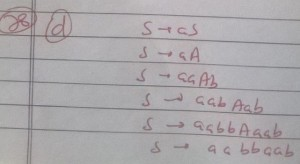# GATE | Gate IT 2008 | Question 76

A CFG G is given with the following productions where S is the start symbol, A is a non-terminal and a and b are terminals.
S→aS∣A
A→aAb∣bAa∣ϵ
For the correct answer in Q75, how many steps are required to derive the string and how many parse trees are there?
(A) 6 and 1
(B) 6 and 2
(C) 7 and 2
(D) 4 and 2

Explanation: